1. Home
2. Docs
3. Numerical Methods
4. Solution of Nonlinear Equations
5. Bisection Method

# Bisection Method

## Introduction

• Simplest method
• Convergent
• Requires initial guesses
• Closed bracket method
• Also known as binary chopping or half interval method

This method is based on the immediate value theorem which states that if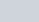is continuous in the interval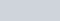and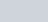andhas different signs then the equation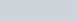has atleast one root between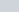and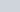.

Here mid-point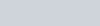This gives us two new intervals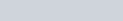.

if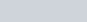thenis the root of. Otherwise, if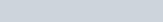then the root lies between&. Then we bisect the interval as before and continue the process until we meet the accuracy as defined.

## Algorithm

1. Define functionand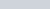2. Guess two initial valuesand3. Computeand4. Ifgoto step 8, otherwise
5. Calculate6. Check if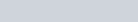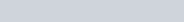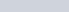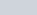goto step 5
7. Print root =8. End

## Psuedocode

FUNCTION Bisect(xl, xu, es, imax, xr, iter, ea)
iter = 0
DO
xrold = xr
xr = (xl 1 xu) / 2
iter = iter 1 1
IF xr ? 0 THEN
ea = ABS((xr 2 xrold) / xr) * 100
END IF
test = f(xl) * f(xr)
IF test , 0 THEN
xu = xr
ELSE IF test . 0 THEN
xl = xr
ELSE
ea = 0
END IF
IF ea < es OR iter >= imax EXIT
END DO
Bisect = xr
END Bisect

## Code

### C Program

float bisection(float x1, float x2, int n){
float x, fx1, fx2;
int i;
for(i = 0; i < n; i++){
x = (x1 + x2)/2;
fx1 = fn(x1);
fx2 = fn(x2);
if(fx1*fx2 < 0){
x1 = x;
}
else{
x2 = x;
}
}
return x;
}

 float fn(float x){
return x*x*x - 2*x - 5;
}

### Javascript

Function for bisection

function bisection(a,b,n,f)
{
var x = (a+b)/2;
var e = Math.pow(10, -n);
var i = 0;
while(Math.abs(f(x))>e)
{
if(f(a)*f(x)<0)
{
b = x;
}
else
{
a = x;
}
x = (a+b)/2;
i++;
console.log(x)
}
return x;
}

The function representing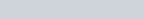function f(x)
{
return x*x*x -2*x - 5;
}
console.log(bisection(2,3,3,f));
Output :

2.25
2.125
2.0625
2.09375
2.109375
2.1015625
2.09765625
2.095703125
2.0947265625
2.09423828125
2.094482421875
2.094482421875

## Convergence

In Bisection method interval is halved in every iteration. After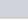iteration size of interval is reduced to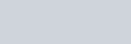Now we can say that maximum error afteriteration is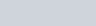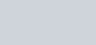Similarly, after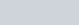iteration maximum error is given by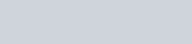This equation shows that error is halved after each iteration of bisection method. Therefore, we can say tat the bisection method converges linearly.